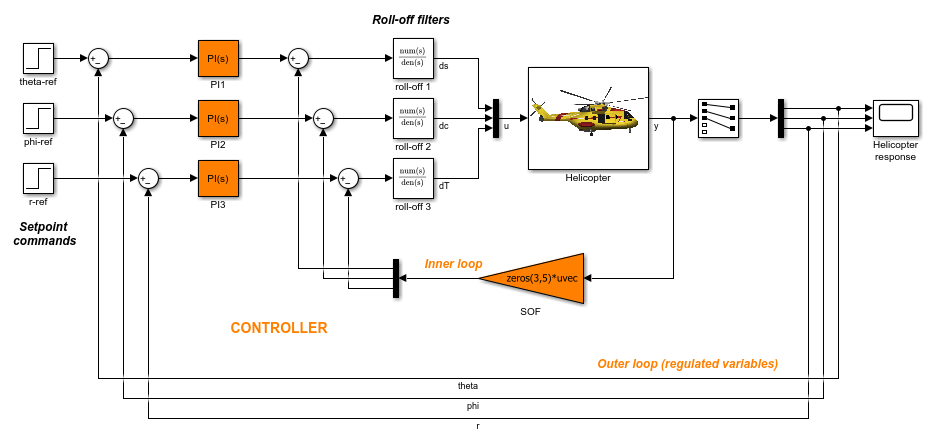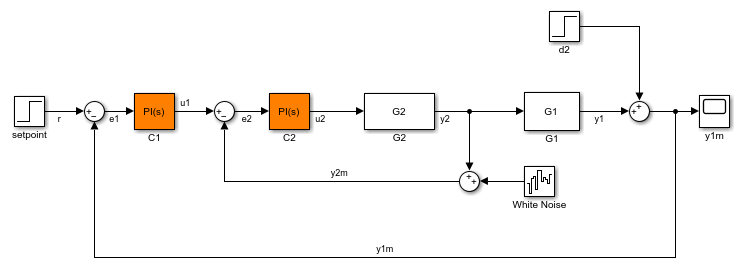# getBlockValue

Get current value of tuned block parameterization in `slTuner` interface

## Syntax

``value = getBlockValue(st,blk)``
``[val1,val2,...] = getBlockValue(st,blk1,blk2,...)``
``S = getBlockValue(st)``

## Description

`getBlockValue` lets you access the current value of the parameterization of a tuned block in an `slTuner` interface.

An `slTuner` interface parameterizes each tuned Simulink® block as a Control Design Block (Control System Toolbox), or a generalized parametric model of type `genmat` or `genss`. This parameterization specifies the tuned variables for commands such as `systune`.

example

````value = getBlockValue(st,blk)` returns the current value of the parameterization of a tunable block, `blk`, in an `slTuner` interface.```

example

````[val1,val2,...] = getBlockValue(st,blk1,blk2,...)` returns the current values of the parameterizations of one or more tuned blocks of `st`.```

example

````S = getBlockValue(st)` returns a structure containing the current values of the parameterizations of all tuned blocks of `st`.```

## Examples

collapse all

Create an `slTuner` interface for the `scdcascade` model.

```open_system('scdcascade') st = slTuner('scdcascade',{'C1','C2'}); ```Examine the current parameterization value of one of the tuned blocks.

```val = getBlockValue(st,'C1') ```
```val = 1 Kp + Ki * --- s with Kp = 0.158, Ki = 0.042 Name: C1 Continuous-time PI controller in parallel form. ```

Create an `slTuner` interface for the `scdhelicopter` model.

```open_system('scdhelicopter') st = slTuner('scdhelicopter',{'PI1','PI2','PI3','SOF'}); ```Retrieve the values of parameterizations for the PI controller blocks in the model.

```[valPI1,valPI2,valPI3] = getBlockParam(st,'PI1','PI2','PI3'); ```

Create an `slTuner` interface for the `scdcascade` model.

```open_system('scdcascade') st = slTuner('scdcascade',{'C1','C2'}); ```Retrieve the parameterization values for both tuned blocks in `st`.

```blockValues = getBlockValue(st) ```
```blockValues = struct with fields: C1: [1x1 pid] C2: [1x1 pid] ```

`blockValues` is a structure with field names corresponding to the names of the tunable blocks in `st`. The field values of `blockValues` are `pid` models, because `C1` and `C2` are both PID Controller blocks.

## Input Arguments

collapse all

Interface for tuning control systems modeled in Simulink, specified as an `slTuner` interface.

Block in the list of tuned blocks for `st`, specified as a character vector or string. You can specify the full block path or any portion of the block path that uniquely identifies the block among the other tuned blocks of `st`.

Example: `blk = 'scdcascade/C1'`, ```blk = "C1"```

## Output Arguments

collapse all

Current value of block parameterization, returned as a numeric LTI model (Control System Toolbox), such as `pid`, `ss`, or `tf`.

When the tuning results have not been applied to the Simulink model using `writeBlockValue`, the value returned by `getBlockValue` can differ from the actual Simulink block value.

### Note

Use `writeBlockValue` to align the block parameterization values with the actual block values in the Simulink model.

Current values of all block parameterizations in `st`, returned as a structure. The names of the fields in `S` are the names of the tuned blocks in `st`, and the field values are the corresponding numeric LTI models.

You can use this structure to transfer the tuned values from one `slTuner` interface to another `slTuner` interface with the same tuned block parameterizations.

```S = getBlockValue(st1); setBlockValue(st2,S);```

collapse all

### Tuned Blocks

Tuned blocks, used by the `slTuner` interface, identify blocks in a Simulink model whose parameters are to be tuned to satisfy tuning goals. You can tune most Simulink blocks that represent linear elements such as gains, transfer functions, or state-space models. (For the complete list of blocks that support tuning, see How Tuned Simulink Blocks Are Parameterized). You can also tune more complex blocks such as SubSystem or S-Function blocks by specifying an equivalent tunable linear model (Control System Toolbox).

Use tuning commands such as `systune` to tune the parameters of tuned blocks.

You must specify tuned blocks (for example, `C1` and `C2`) when you create an `slTuner` interface.

`st = slTuner('scdcascade',{'C1','C2'})`

You can modify the list of tuned blocks using `addBlock` and `removeBlock`.

To interact with the tuned blocks use:

### Tuned Variables

Within an `slTuner` interface, tuned variables are any Control Design Blocks (Control System Toolbox) involved in the parameterization of a tuned Simulink block, either directly or through a generalized parametric model. Tuned variables are the parameters manipulated by tuning commands such as `systune`.

For Simulink blocks parameterized by a generalized model or a tunable surface:

For Simulink blocks parameterized by a Control Design Block, the block itself is the tuned variable. To modify the block value, you can use either `setBlockValue` or `setTunedValue`. Similarly, you can retrieve the block value using either `getBlockValue` or `getTunedValue`.